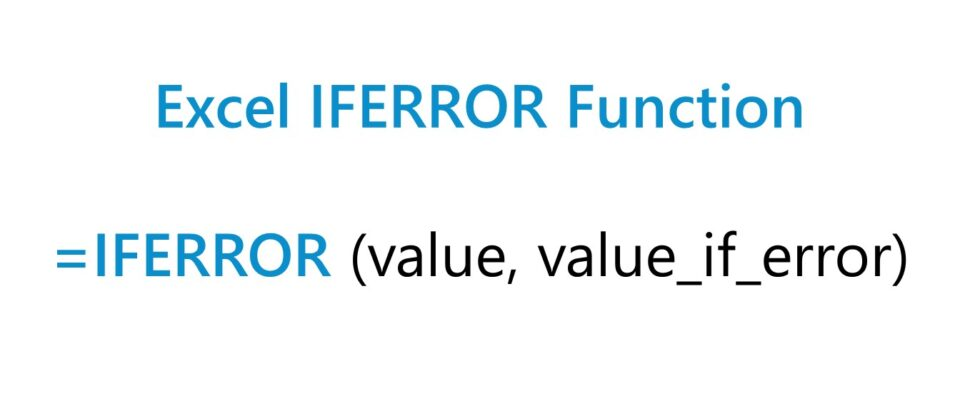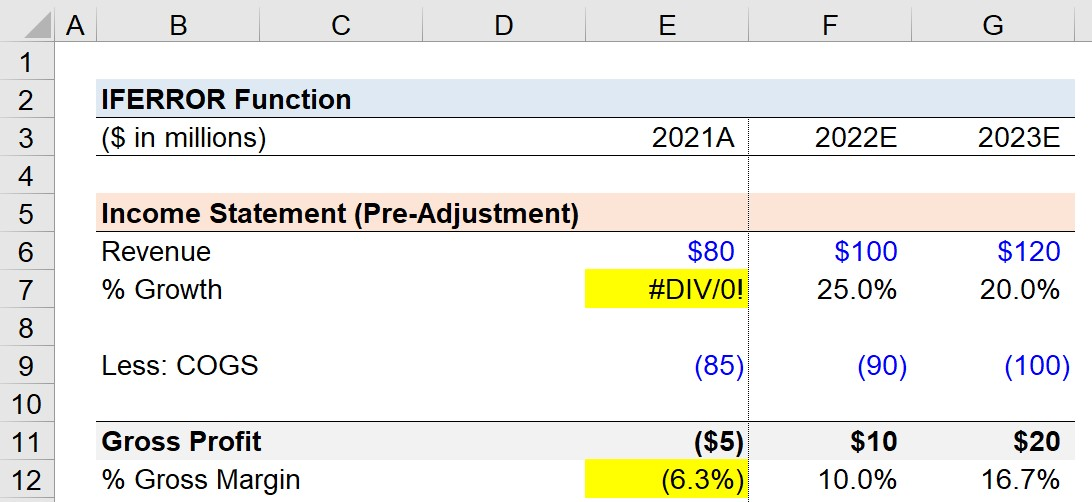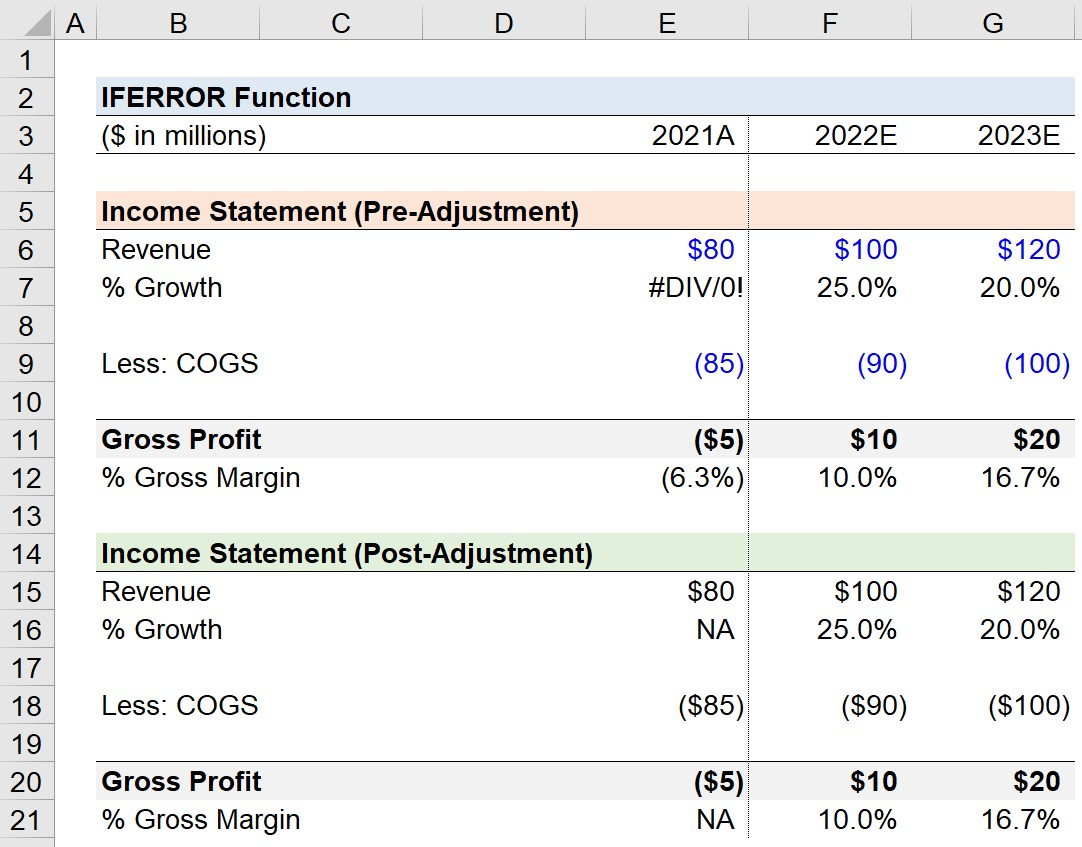Welcome to Wall Street Prep! Use code at checkout for 15% off.# IFERROR Function

Guide to Understanding the “IFERROR” Function in Excel## How to Use IFERROR Function in Excel (Step-by-Step)

The Excel IFERROR function is utilized to identify and prevent error messages from appearing in a spreadsheet.

Using the IFERROR function is one method to ensure that errors within a financial model are brought to the attention of the user with a custom value.

Some of the most common error messages that trigger the “IFERROR” function are the following types:

• #VALUE!”: One of the more general error messages that occurs when one of the values in a formula contains an inconsistency, such as attempting to add a cell containing text as part of a numerical calculation.
• #REF!”: The cell reference is not valid, which is often a result of a cell that was formerly a part of a calculation that is accidentally deleted or moved elsewhere.
• #DIV/0!”: The formula is attempting to divide a number by zero.
• #NUM!”: The formula cannot be calculated due to limitations or errors. A multitude of problems could be the source of the problem, but frequently it is related to the calculation output being either too small or too large.
• #NAME?”: The syntax in the formula contains an unrecognized cell reference or named cell (or range), or maybe quotations are missing.
• #NULL!”: The formula involves a cell that contains an empty value, or an accidental space.

In practice, the most common custom returned value is “NA”, “N/A” or “n.a.”, which refers to the phrase “not applicable”.

The general rule of thumb is that the returned value should be in the form of text, as opposed to a number.

For example, if the returned value is the number “0”, a mistake could easily be made where the cell containing the error is included in a calculation with an actual numerical value.

## Excel IFERROR Function Formula Syntax

The formula for using the IFERROR function in Excel is as follows.

=IFERROR(value, value_if_error)
• value” → The formula that the function checks to confirm there is no error.
• value_if_error” → The custom returned value if an error is identified by Excel.

If there is no error, the calculation in the first input is performed as normal, otherwise, the error message is shown (and the error is “trapped”).

## IFERROR Function Calculator — Excel Model Template

We’ll now move on to a modeling exercise, which you can access by filling out the form below.Submitting...

## Step 1. Income Statement Assumptions

Suppose we’re in the initial stages of building an income statement forecast.

The financials for the company—from revenue (the “top line”) to the gross profit line item—are as follows.

Income Statement 2021A 2022E 2023E
Revenue \$80 million \$100 million \$120 million
Less: COGS (\$85 million) (\$85 million) (\$85 million)
Gross Profit (\$5 million) \$10 million \$20 million

## Step 2. “#DIV/0!” Error Message

In the next step, we’ve added a line item to calculate the year-over-year growth rate (YoY) of the company’s revenue.

We’ll calculate the YoY growth rate by taking the current year revenue, dividing it by the prior year revenue, and subtracting one from the result.

• Revenue Growth YoY, 2021A = #DIV/0!
• Revenue Growth YoY, 2022E = 25.0%
• Revenue Growth YoY, 2023E =20.0%

However, there is no historical data for Year 0 (2021A), so an “#DIV/0!” error message appears.In order to prevent our model from showing the error message, we’ll wrap our YoY growth formula with the following “IFERROR” function.

=IFERROR(E15/D15-1,”NA”)

## Step 3. Manual Error Message Catching in Financial Models

In the final part of our quick lesson, we’ll show an example of an error that is not necessarily an “error”, per se, to Excel.

Here, we’ve calculated the gross profit for each period, so we can determine the gross margin by dividing the gross profit by the revenue in the corresponding year.

• Gross Margin, 2021A = (6.3%)
• Gross Margin, 2022E = 10.0%
• Gross Margin, 2023E =16.7%

The outlier is Year 0 (2021A), since the gross margin is a negative figure, which is clearly an “error” yet Excel would not recognize it as such.

Therefore, we’ll enter the following formula to handle the error manually.

=IF(E20/E15<0,”NA”,E20/E15)The formula states that if the gross margin is less than zero, then return the “NA” error message.

If the gross margin is greater than zero, however, the calculated gross margin should be returned as usual, as performed in the next two periods.

In cases such as the example shown here, “NM” or “N/M” can be used as the returned error message, which refers to the phrase “not meaningful”.

For the most part, ensuring there are no calculations that are unreasonable (or not plausible) in a model results in a cleaner, more intuitive financial model.Other instances where these issues can require a manual entry “IF” function to catch an error would be in the following scenarios:

• “Unbalanced” Balance Sheet: Most balance sheets in 3-statement financial models contain a “Check” row to confirm the balance sheet is in fact “balanced”, i.e. the accounting equation is true, wherein the sum of the company’s liabilities and equity equals its assets.
• Revolver Non-Compliance: In a debt schedule, the revolving credit facilities, i.e. “revolvers”, must be modeled with the borrowing limit in mind. If the company borrowing needs to exceed the total capacity (i.e. the upper limit), an “IF” function can be used to show an error message such as “Non-Compliant” or “Overdrawn”.
• Negative Pre-Tax Income: A financial model could mistakenly tax-affect the negative earnings of an unprofitable company with no taxable income. For companies that are either unprofitable or barely profitable, the recommendation is to wrap the income tax provision line with an “IF” function that first confirms that the pre-tax income of the company is positive.
Turbo-charge your time in Excel Used at top investment banks, Wall Street Prep's Excel Crash Course will turn you into an advanced Power User and set you apart from your peers.